2011-11-30 15:22:49 honesty2008 阅读数 3092
• ###### MATLAB图像处理

全面系统的学习MATLAB在图像处理中的应用

20277 人正在学习 去看看 魏伟①找到亮度的一阶导数在幅度上比指定的阈值大的地方
②找到亮度的二阶导数有零交叉的地方。

本实验完成方法很多，可以通过以下途径解决：①直接用edge函数
②用imfilter 模拟③直接进行模拟 。值得注意的是由于Roberts掩膜是2*2的,

% 代码一 直接通过edge函数检测
clear;clc;figure;
subplot(2,2,1);imshow(I);title('orignal');
I1=edge(I,'sobel');
subplot(2,2,2);imshow(I1);title('sobel');
I2=edge(I,'prewitt');
subplot(2,2,3);imshow(I2);title('prewitt');
I3=edge(I,'roberts');
subplot(2,2,4);imshow(I3);title('roberts');
% 代码二 直接模拟
clear;clc;figure;
subplot(2,2,1);imshow(I);title('orignal');
s1=[-1 -2 -1; 0 0 0; 1 2 1];
s2=[-1 0 1; -2 0 2; -1 0 1 ];
p1=[-1 -1 -1; 0 0 0 ;1 1 1];
p2=[-1 0 1;-1 0 1; -1 0 1 ];
r1=[-1 0 ;0 1];r2=[0 -1; 1 0];
[r,c]=size(I);I=double(I);
for u=1:r-2
for v=1:c-2
gx=sum(sum(I(u:u+2,v:v+2).*s1));
gy=sum(sum(I(u:u+2,v:v+2).*s2));
Is(u,v)=sqrt(gx*gx+gy*gy);
gx=sum(sum(I(u:u+2,v:v+2).*p1));
gy=sum(sum(I(u:u+2,v:v+2).*p2));
Ip(u,v)=sqrt(gx*gx+gy*gy);
gx=sum(sum(I(u+1:u+2,v+1:v+2).*r1));
gy=sum(sum(I(u+1:u+2,v+1:v+2).*r2));
Ir(u,v)=sqrt(gx*gx+gy*gy);
end
end

Is1=Is;Ip1=Ip;Ir1=Ir;
scale=0.4;
Is2=Is1>max(max(Is1))*scale;
Ip2=Ip1>max(max(Ip1))*scale;
Ir2=Ir1>max(max(Ir1))*scale;
subplot(2,2,2);imshow(Is2,[]);title('sobel');
subplot(2,2,3);imshow(Ip2,[]);title('prewitt');
subplot(2,2,4);imshow(Ir2,[]);title('roberts');2018-10-03 15:46:39 u013921430 阅读数 4193
• ###### MATLAB图像处理

全面系统的学习MATLAB在图像处理中的应用

20277 人正在学习 去看看 魏伟

## 灰度图边缘检测

在学习图像处理时，首先接触到的就是灰度图像的边缘检测，这是图像处理最基础的也是最重要的一环，熟悉图像边缘检测有助于我们学习其他的数字图像处理方法。由于图像的边缘区域会存在明显的像素值阶跃，因此边缘检测主要是通过获得图像灰度梯度，进而通过梯度大小和变化来判断图像边缘的。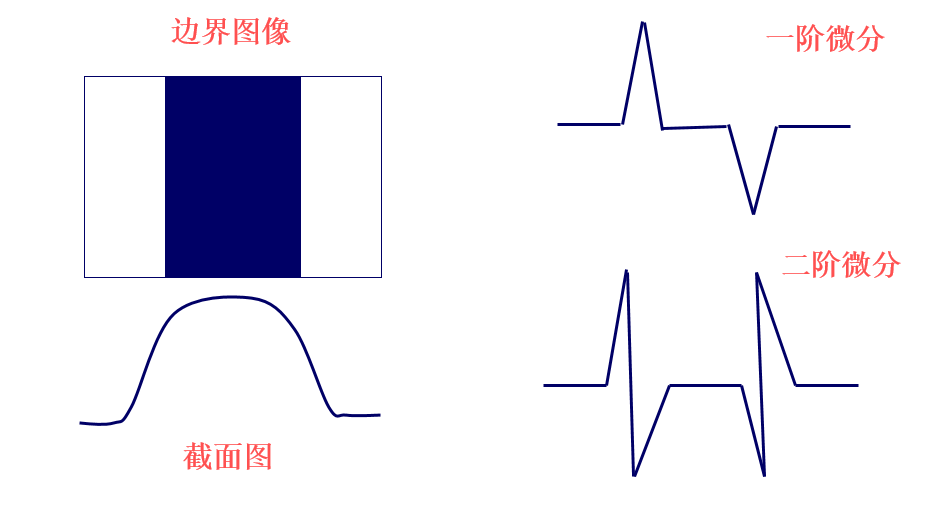因此，我们可以通过一阶差分；
$\Delta f_{x}(x,y)=f(x+1,y)-f(x,y)\\ \Delta f_{y}(x,y)=f(x,y+1)-f(x,y)$
或者二阶差分对边缘区域进行判断；
$\Delta f_{xx}(x,y)=f(x+1,y)+f(x-1,y)-2f(x,y)\\ \Delta f_{yy}(x,y)=f(x,y+1)+f(x,y-1)-2f(x,y)$
其中一阶差分可以判断边缘是否存在，二阶差分还可以根据正负号判断像素点在图像边缘亮的一侧还是暗的一侧。
其他的边缘检测方法还包括一些梯度算子，例如Prewitt算子、Sobel算子，Canny算子，LOG边缘检测算子等，在此不做说明。

## 彩色图边缘检测

RGB 图像使用三个通道存储像素信息，我们可以将这三个通道的信息看作是一个矢量，而矢量是不存在梯度的概念的，我们无法直接将上诉方法或算子直接用于RGB 图像，而且RGB图像单个通道的梯度信息又无法反映整体的梯度信息。
在《数字图像处理》（冈萨雷斯）中提到了一种针对彩色图像的边缘检测方法，这种方法由 Di Zenzo 等人在1986年提出，下面就一起看看这种方法如何得出。

在图像多通道图像$f(x,y)$ 中的某一点$P(x,y)$ 处，假设其梯度方向为$\theta$
$\Delta f=\left \| f(x+\varepsilon cos\theta,y+\varepsilon sin\theta) -f(x,y)\right \|$
为了便于计算，将计算绝对值换为计算平方，令
$\Delta f^{2}=\left \| f(x+\varepsilon cos\theta,y+\varepsilon sin\theta) -f(x,y)\right \|^{2}$
对$f(x+\varepsilon cos\theta,y+\varepsilon sin\theta)$进行二元泰勒展开；
\begin{aligned} f(x+\varepsilon cos\theta,y+\varepsilon sin\theta)&=f(x,y)+\sum_{i=1}^{m}(\varepsilon cos\theta\cdot\frac{\partial f_{i}(x,y)}{\partial x} +\varepsilon sin\theta\cdot\frac{\partial f_{i}(x,y)}{\partial y} )+o^{n}\\ &\approx f(x,y)+\sum_{i=1}^{m}(\varepsilon cos\theta\cdot\frac{\partial f_{i}(x,y)}{\partial x} +\varepsilon\cdot sin\theta\cdot\frac{\partial f_{i}(x,y)}{\partial y} ) \end{aligned}
其中$m$表示图像通道数目，为了方便表述使用$\frac{\partial f_{i}}{\partial x}$代替$\frac{\partial f_{i}(x,y)}{\partial x}$，而在求导时各个通道之间是相互独立的，则有；
$\Delta f^{2}\approx\sum_{i=1}^{m}(\varepsilon cos\theta\cdot\frac{\partial f_{i}}{\partial x} +\varepsilon sin\theta\cdot\frac{\partial f_{i}}{\partial y} )^{2}$
重新定义一个函数$G(\theta)$，令
\begin{aligned} G(\theta)&=\sum_{i=1}^{m}(\varepsilon cos\theta\cdot\frac{\partial f_{i}}{\partial x} +\varepsilon sin\theta\cdot\frac{\partial f_{i}}{\partial y} )^{2}\\ &=\varepsilon ^{2}(cos\theta^{2}\sum_{i=1}^{m}\left \|\frac{\partial f_{i}}{\partial x}\right \|^{2}+sin\theta^{2}\sum_{i=1}^{m}\left \|\frac{\partial f_{i}}{\partial y}\right \|^{2}+2sin\theta cos\theta \sum_{i=1}^{m}\frac{\partial f_{i}}{\partial x}\frac{\partial f_{i}}{\partial y}) \end{aligned}
进一步舍去式子中的$\varepsilon$ 项，令
$G(\theta)=cos\theta^{2}\sum_{i=1}^{m}\left \|\frac{\partial f_{i}}{\partial x}\right \|^{2}+sin\theta^{2}\sum_{i=1}^{m}\left \|\frac{\partial f_{i}}{\partial y}\right \|^{2}+2sin\theta cos\theta \sum_{i=1}^{m}\frac{\partial f_{i}}{\partial x}\frac{\partial f_{i}}{\partial y}$
为了进一步方便表述；令
$E=\sum_{i=1}^{m}\left \| \frac{\partial f_{i}}{\partial x} \right \|^{2}; F=\sum_{i=1}^{m}\left \|\frac{\partial f_{i}}{\partial y}\right \|^{2}; H=\sum_{i=1}^{m}\frac{\partial f_{i}}{\partial x}\frac{\partial f_{i}}{\partial y}$
$G(\theta)=cos\theta^{2}E+sin\theta^{2}F+2sin\theta cos\theta H$
现在$\theta$成为了式子中唯一的变量，再回到边缘的定义上，边缘的方向是图像像素梯度最大的方向。也就是说梯度的方向$\theta_{max}$ 会使$G(\theta)$ 取最大值，则；
$\theta_{max}=\underset{argmax}{G(\theta )}$
对$G(\theta)$ 进行求导；
$G(\theta )^{'}=-Esin2\theta +F sin2\theta+2 H cos2\theta$
令$G(\theta )^{'}=0$，得；
$tan ~2\theta_{max} =\frac{2H}{E-F}\\ \theta_{max}=\frac{1}{2}arctan(\frac{2H}{E-F}+k\pi)$
很明显$G(\theta )$ 是一个以$\pi$ 为周期的周期函数，如果只考虑区间$\left [ 0 ,\pi\right )$，且$\theta_{max}$ 落到该区间内，则会有另一个让$G(\theta )$取极值的解也落在该区域内，这个值是$\theta_{max}+ \frac{\pi}{2}$或者$\theta_{max}-\frac{\pi}{2}$。但是不论如何这两个解有一个让$G(\theta )$取极大值，另一个让其取极小值，两个角度相差 90°。

说到这里大家应该都明白了，两个角度对应的方向是相互垂直的，一个是垂直于边缘的方向，也就是边缘的法向，此时梯度取最大值。另一个是平行于边缘的方向，是边缘的切向，此时梯度取极小值。

### RGB图像的边缘检测

在RGB图像中，$m=3$，再令；
$\overset{\rightarrow }{u}=\frac{\partial R}{\partial x}\overset{\rightarrow }{r}+\frac{\partial G}{\partial x}\overset{\rightarrow }{g}+\frac{\partial B}{\partial x}\overset{\rightarrow }{b}$
$\overset{\rightarrow }{v}=\frac{\partial R}{\partial y}\overset{\rightarrow }{r}+\frac{\partial G}{\partial y}\overset{\rightarrow }{g}+\frac{\partial B}{\partial y}\overset{\rightarrow }{b}$
其中$\overset{\rightarrow }{r}$$\overset{\rightarrow }{g}$$\overset{\rightarrow }{b}$分别代表不同颜色分量的单位向量，则
$g_{xx}=E=\overset{\rightarrow }{u}^{\tiny{T}}\overset{\rightarrow }{u}=\left \| \frac{\partial R}{\partial x} \right \|^{2}+\left \| \frac{\partial G}{\partial x} \right \|^{2}+\left \| \frac{\partial B}{\partial x} \right \|^{2}$
$g_{yy}=F=\overset{\rightarrow }{v}^{\tiny{T}}\overset{\rightarrow }{v}=\left \| \frac{\partial R}{\partial y} \right \|^{2}+\left \| \frac{\partial G}{\partial y} \right \|^{2}+\left \| \frac{\partial B}{\partial y} \right \|^{2}$
$g_{xy}=H=\overset{\rightarrow }{u}^{\tiny{T}}\overset{\rightarrow }{v}=\frac{\partial R}{\partial x}\frac{\partial R}{\partial y}+\frac{\partial G}{\partial x}\frac{\partial G}{\partial y}+\frac{\partial B}{\partial x}\frac{\partial B}{\partial y}$
在利用Di Zenzo 提出的方法求得$\theta_{max}$ 后，将以上符号带入到$G(\theta)$，可以计算出像素点梯度大小为
$G(\theta)=\left \{ \frac{1}{2}\left [ (g_{xx}+g_{yy}) +(g_{xx}-g_{yy})cos~2\theta_{max} +2g_{xy}sin~2\theta_{max} \right ]\right \}^{\frac{1}{2}}$
进而可以根据梯度大小进行边缘检测。

## 参考资料

1. S. Di Zenzo, A note on the gradient of a multi-image, Computer Vision, Graphics, and Image Processing 33 (1) (1986) 116–125.
2. 《数字图像处理》 (冈萨雷斯)

2018-04-18 20:02:11 Gentleman_Qin 阅读数 7801
• ###### MATLAB图像处理

全面系统的学习MATLAB在图像处理中的应用

20277 人正在学习 去看看 魏伟

## 高光谱图像处理之目标检测技术

1、高光谱图像目标检测的发展趋势和研究现状：

20世纪80年代末，美国的一些研究机构开始利用高光谱图像数据进行目标检测方面的研究。自上世纪九十年代，国外出现了进行高光谱图像目标检测算法理论研究的研究组。由Reed和Yu提出了基于广义似然比检验的恒虚警RX 检测器(RXD)。Chang课题组提出了基于正交子空间投影的OSP检测方法， Harsanyi提出了基于约束能量最小化的CEM算法。未来高光谱目标检测的发展将会越来越重视实用性，算法的性能将会进一步提高，同时更也加适合使用FPGA硬件对其进行加速从而具有更高的实际应用价值。

2、高光谱目标检测技术的应用范围：

高光谱目标检测具有较强的实用性，可应用于公共安全、环境检测、城市规划、食品卫生、地质岩矿的识别等众多方面。图1.1 高光谱图像数据结构

3、高光谱图像数据的特点：

高光谱图像数据“图谱合一”,具有丰富的光谱维信息。高光谱图像数据共有三个维度，如图2.1所示，其中，图像空间维信息x、y用于表示物体的实际空间分布，而光谱波段L用于表示每个像素的光谱属性。

4、高光谱图像目标检测原理：

高光谱图像的各波段在成像范围内都是连续成像，因此高光谱的光谱曲线一般是平滑的、连续的曲线。高光谱图像的波段L中涵盖了物质的光谱信息，而每种物质的光谱信息都不一样，我们可以利用图像像素的光谱波段L所包含的特定的光谱信息来判断该像素所代表的特定的物质种类。

5、高光谱图像目标检测流程：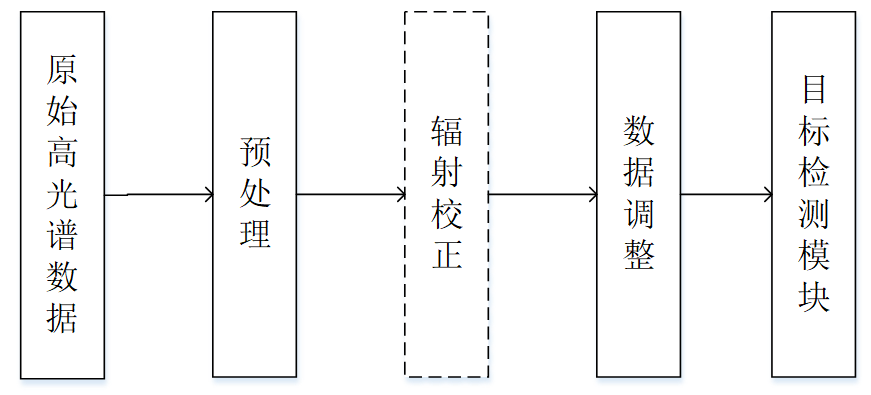图1.2 高光谱图像目标检测流程图

如图所示，对于拍摄得到的原始高光谱图像数据，需要先对数据进行预处理，包括数据格式化、无用数据剔除以及亮度到反射率的转化等。同时，对于遥感仪拍摄的高光谱图像还需要进行辐射校正，在目标检测前，应对数据进行调整，包括数据归一化等。最后根据已知的先验信息选择相应的目标检测算法进行检测。

6、现场可编程门阵列（FPGA）在高光谱图像处理中的应用：

一个FPGA可以大致定义为一系列互连的逻辑块。这些器件的主要优点之一是，为了实现不同的组合或时序逻辑功能，可以根据需要多次重新配置逻辑块及其互连。这一特性为FPGA提供了软件和硬件系统的优势。因为FPGA具有比专用集成电路（ASIC）更多的灵活性和更短的开发时间，更接近GPU提供的性能，但同时功耗要比GPU低得多。FPGA供应商通过改进FPGA体系结构（包括优化的硬件模块）并利用最新的硅技术不断提升FPGA的功耗和能效。可重构性、低功耗的特点以及FPGA对空间电离辐射耐受性的提高，这些因素已经使得FPGA成为目前板载高光谱遥感的最佳选择之一。

1、方法分类：

高光谱目标检测方法按照先验信息是否已知分为监督方法和非监督方法。前者用于目标光谱已知的情况下，利用目标光谱与图像像元光谱进行匹配，从而完成目标检测，比如CEM算法、OSP算法；后者多用于异常目标检测，一般不需要目标和背景的先验信息，根据高光谱图像数据获取目标检测所需要的数据，然后根据数据的大小来判断是否为异常目标，比如RXD算法。

2、CEM、OSP、RXD算法的区别：

（1）CEM（Constrained Energy Minimization）算法：

CEM算法主要思想是设计一个FIR线性滤波器，使得在满足约束条件式的情况下滤波器的输出能量最小。该算法不需要图像的背景信息，只需要知道要检测的先验光谱信息（目标向量）即可，具体方法是通过高光谱图像数据和先验已知的待检测目标确定一个滤波向量，让图像经过该滤波向量即可得到检测结果，其中滤波向量的作用是滤除图像中的非目标像素，让感兴趣的目标能够通过，同时抑制由其他信号带来的滤波器输出能量。

（2）OSP（Orthogonal Subspace Projection）算法：

OSP算法与CEM算法相比，最大区别在于不仅需要目标的先验知识，还需要图像中背景的先验知识，但在实际中中这些先验信息很难全部得到。在高光谱检测中我们一般用其来检测异常。该算法需要前提条件：图像信息、目标像元、非目标像元（异常目标）信息。

（3）RXD(Reed-XiaoliDetector)算法：

RXD算法是异常目标检测领域中最基础的算法，不需要目标光谱的先验知识，而是基于背景服从多元正态分布的假设，通过检测与背景分布中心相比属于异常像元，并在这些感兴趣区域进一步查找可能存在的目标。该算法主要针对的是小目标检测问题。

（4）确定所采用的目标检测算法：

由于我们的应用场景多为有特定目标的目标检测，CEM正是针对未知场景中可能存在的特定目标的检测，只需要知道目标的光谱信息即可，而RXD算法适应于对特定场景的异常（未知目标）检测，而OSP算法除了需要已知目标光谱还需要背景信息。综上，我决定采用CEM算法进行高光谱目标检测的实现。

1、算法步骤

（1）对高光谱图像进行预处理，得到二维化和归一化后的数据r（L*N）；

（2）根据图像数据r，求得图像的自相关矩阵：（3）确定目标光谱向量dd大小为L*1）；

（4）根据公式：设计FIR线性滤波器：（5）将归一化后的数据经过FIR滤波器，得到输出信号y2、问题分解：

CEM算法的实现可分为三部分：自相关矩阵、矩阵求逆、线性FIR滤波器。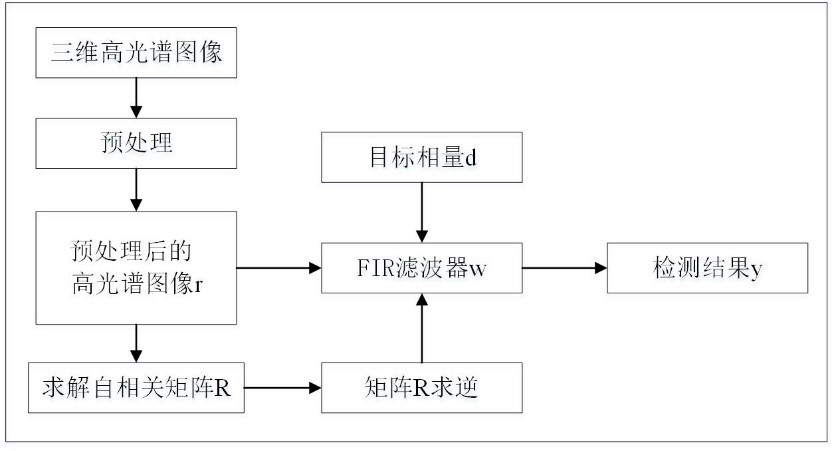如图所示，首先根据高光谱图像r求得自相关矩阵，再利用矩阵求逆模块求得自相关矩阵的逆矩阵，结合从光谱库获取到的目标向量的先验信息求得FIR滤波器的滤波向量，最后将高光谱图像r通过FIR滤波器即可得到最终的检测结果。

1、算法流程：

在MATLAB和C语言中实现CEM算法的具体流程如图所示，因为语言特性是串行执行命令，所以在编写程序时与硬件设计比较更加直接明了。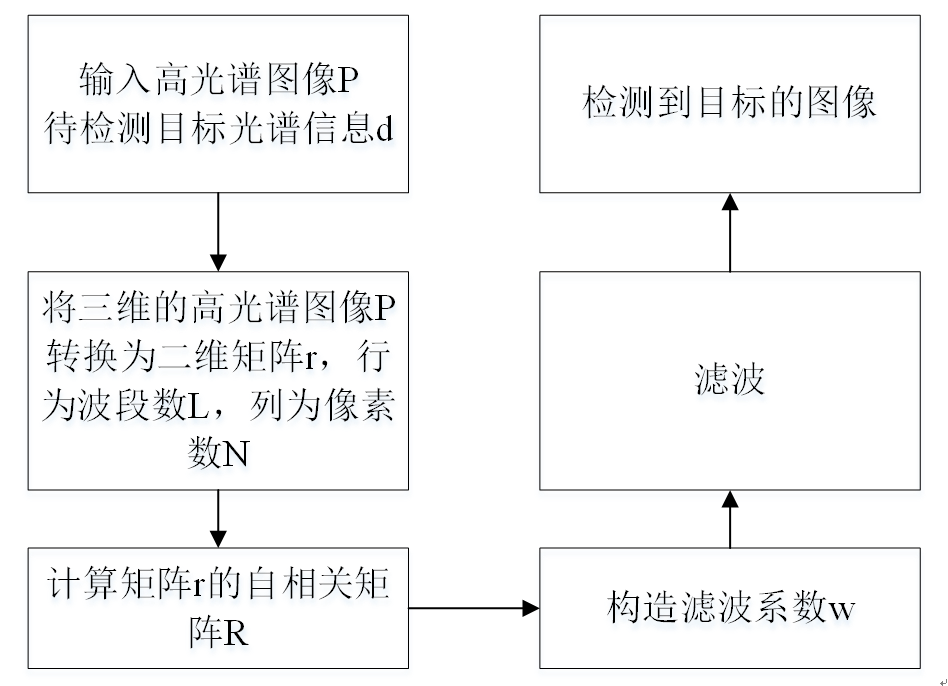2、数据预处理：

对前期得到的高光谱图像在MATLAB平台上进行预处理。这一过程主要对原始的200*200*189大小的高光谱图像进行操作：

（1）二维化：通过调用MATLAB里面的reshape（）函数实现。

（2）归一化：采用“min-max”方法。 具体步骤是先找到数据的最大值
（maxA）和最小值（minA）， 通过 y = (x-minA) /(maxA-minA) 计算的 y 即为归一化后的数据。高光谱数据量大，也造成了数据的存储比较困难，数据在程序中的存储等处理

3、CEM算法的MATLAB实现：

首先，根据 2 对高光谱图像数据进行预处理，而后求其自相关矩阵。高光谱图像数据的大小为200*200*189，其中200*200为像素数，189为波段数。首先用reshape()函数和transpose()函数将200*200*189的三维高光谱图像数据转为189*40000的二维矩阵，再用自己写的Normalize()函数对矩阵数据用“min-max”方法进行归一化，然后根据公式式利用MATLAB中的矩阵求转置函数transpose()和矩阵相乘操作得到一个189*189的矩阵，最后对189*189的矩阵除以像素数N即可得到自相关矩阵。

4、CEM算法的C语言实现：

在Visual Studio平台上完成CEM算法C程序的编写。相比于MATLAB实现，CEM算法的C语言实现主要难点在于矩阵运算。因为在C语言中，矩阵的转置、相乘、求逆等操作均没有现有的函数，需要编写相应的函数。在实现矩阵基本运算过程中,通过动态分配内存运用二维指针传递参数,完成矩阵加减法运算、矩阵转置运算、矩阵相乘运算以及矩阵求逆运算，这样可以节省存储空间，使用完后释放空间即可。

CEM算法的C语言实现主要包括如下步骤：

（1）在MATLAB中，将归一化后的高光谱图像数据转为189*40000的二维形式，保存为CEM.mat；

（2）编写矩阵初始化、矩阵转置、矩阵相乘、矩阵求逆、内存释放函数；

（3）编写main函数，读CEM.mat，调用上述函数，求得FIR滤波器，进而获得输出信号y，将其写入CEM.txt；

（4）在MATLAB中显示CEM.txt中数据所代表的图像。

5、难点解决：

（1）矩阵转置

矩阵转置函数的输入是大小为m*n的矩阵A，输出是大小n*m为的矩阵B。采用嵌套的for循环分别遍历矩阵的行和列，将输入的二维矩阵A按列读出，重新按行写入新矩阵B中，即可实现矩阵的转置。

（2）矩阵相乘

矩阵相乘函数的输入是大小m*n为的矩阵A和n*k的矩阵B，输出是大小为m*k的矩阵C。采用嵌套的for循环分别遍历矩阵的行和列，首先将矩阵A按行读出，矩阵B按列读出，然后将读出的矩阵A的第i行和矩阵B的第j列对应位相乘求和，将计算的结果写入矩阵C第i行第j列元素中。

（3）矩阵求逆——QR分解求逆

QR分解求逆的原理是：对于可逆矩阵A，首先利用QR分解将A矩阵分解为Q矩阵和R矩阵。即A=QR，其中Q是正交矩阵，R是上三角矩阵。然后将公式左右同时求逆，可以求得A的逆矩阵，其中Q矩阵的逆矩阵和转置矩阵相同，R求逆有特定的公式。QR分解求逆的运算较为简单，且数据稳定度较高，可以得到误差较小的求逆结果。

6、算法实现结果和对比分析：

1）MATLAB和C语言实现CEM算法的检测结果如图所示：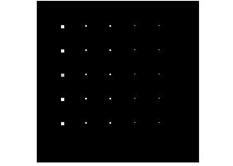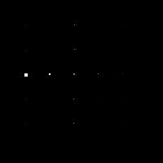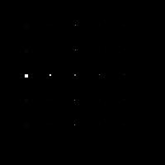（a）               （b）            （c）

######### 转载请注明出处：https://blog.csdn.net/Gentleman_Qin/article/details/79992314 #########

2019-09-30 21:35:38 qq_41413256 阅读数 54
• ###### MATLAB图像处理

全面系统的学习MATLAB在图像处理中的应用

20277 人正在学习 去看看 魏伟

# 目标检测（object detection）

## 目标检测的目的

1. 分类（classification）:假定给定一幅图像或者一段视频，要判断里面有什么类别的目标。
2. 定位（location）:定位出目标的位置，一般用边框将物体圈出来。
3. 检测（detection）:定位出目标的位置并检测出目标是什么。

## 传统的目标检测方法

2. HOG+SVM用于行人检测，通过对行人目标候选区域提取HOG特征，结合SVM分类器进行判定。
3. DPM：基于HOG特征检测的一个变种，该方法是非深度学习方法中检测效果最好，性能最优的。

## 基于深度学习的目标检测

1. One-stageYOLO和SSD系列）：直接回归初目标框位置，不用产生候选框。
2. Two-stageFaster RCNN系列）：利用RPN网络对候选框进行推荐。
上面写了一大堆，其实我也不是很清楚要做什么，这只是一个概述，方便我今后学习用的。就酱

2017-09-22 19:41:43 u011271038 阅读数 528
• ###### MATLAB图像处理

全面系统的学习MATLAB在图像处理中的应用

20277 人正在学习 去看看 魏伟

# 原理

## 梯度算子其中，## Roberts算子## Prewitt算子## Sobel算子## canny检测

1)用高斯平滑处理
2)算出梯度和梯度方向
3)对梯度值进行非极大值抑制
4)用双阈值算法检测和连接边缘